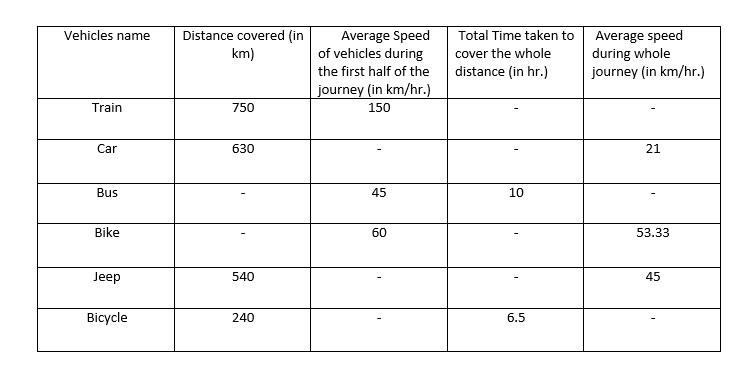# Data Interpretation for SBI PO 2017

Directions (1 – 5): Study the line graph and answer the given question.1. If the profit of company P and Q is same in the year 2012 then the profit of Company Q in the year 2014 is what % of the profit of Company P in the year 2013 ?
a) 43.25
b) 41.75
c) 43.75
d) 41.50
e) 45.25
2. If the ratio between profit earned by Company P and Company Q in the year 2012 and 2014 is  15 : 19 respectively then find the ratio of profit earned by Company P in the year 2013 and Company Q in the same year.
a) 63 : 95
b) 95 : 53
c) 78 : 29
d) 29 : 78
e) 51 : 47
3. Find the avearge profit % increase earned by company Q in given first half of decade .
a) 21.5
b) 22.6
c) 23.5
d) 25.9
e) Data Inadequate
4. If in the year 2011 the profit earned by company P and company Q is 1984 lakhs and 1886 lakhs respectively then find the difference between the profit of company P and company Q in the year 2010.
a) 820 lakhs
b) 600 lakhs
c) 560 lakhs
d) 700 lakhs
e) 500 lakhs
5. If the expenditure of company P and Q in 2015 are equal and the ratio of expenditure and income of P and Q in 2015 are 5 : 6 and 4 : 5 respectively .Find the ratio between the income of P and Q in 2016 respectively if the expenditure of 2015 and 2016 are same for both company.
a) 112 : 125
b) 135 : 157
c) 137 : 178
d) 128 : 117
e) Data Inadequate
Directions (6 – 10): Study the following table carefully and answer the given question.6. If the ratio of time taken by car in first half and second half of journey is 3:7 then find the speed of car during the first half of journey.(in km/hr)
a) 30
b) 32
c) 35
d) 40
e) Can’t be determined
7. If total time taken by train is 8 hours to complete journey then find the average speed of train during second half of the journey.
a) 26.88
b) 32.78
c) 45.24
d) 68.18
e) 45.15
8. If the time taken by bike to cover the second half of journey is half of the time taken by bus to cover the whole distance then find the total distance travelled by bike.
a) 240
b) 480
c) 530
d) 450
e) 650
9. If the speed of jeep in second half of journey is 30 km/hr. then find the speed of first half of journey by jeep.
a) 90 kmph
b) 60 kmph
c) 50 kmph
d) 45 kmph
e) 65 kmph
10. If the average speed of Bus is 56 km/hr. then find the average distance covered by all the vehicles except bike.
a) 544 km
b) 444 km
c) 333 km
d) 244 km
e) 504 km
Solutions:
1. C) Required Average = [(x × 75/100 × 70/100)/(x × 120/100)] × 100 = 43.75%
2. A) Required Ratio = 15/100 × 120 : 19/70 × 100 = 63 : 95
3. E) Data Inadequate
4. D) Required Difference = 1984/124 × 100 – 1886/82 × 100 = 1600 – 2300 = 700 lakhs
5. D) In 2015 for company P,
E : I =( 5 : 6 )×4= 20 : 24
In 2015 for company Q,
E : I = (4 : 5 )×5= 20 : 25
profit of company P in 2016= 4 × 140/100 = 5.6
profit of company Q in 2016= 5 × 68/100 = 3.4
Ratio of income of company P and company Q=25.6 : 23.4=128 : 117
6. C) Total time = 630/21 = 30 hr
Time taken by car in first half = 30/10 × 3 = 9hr
Speed of car in first half = 315/9 = 35 km
7. D) time taken by train in first half of journey=375/150=2.5 hr.
remaining time= 8- 2.5 =5.5 hr,
Required average speed =375/5.5=68.18 km/hr
8. B) since first half and second half is same hence and let speed of bike to cover the second half of journey ,hence average speed
=> 2xy/(x + y) = 53.33 => (2 × 60 × y)/(60 + y) = 53.33 => y = 48
now total distance= 2 ×48×5=480 km
9. A) total time taken by jeep=540/45=12 hr.
now,
T1 + T2 = 12 => 270/S1 + 270/30 = 12 => S1 = 90
Speed during the first half of the journey = 90 km/hr.
10. A) Average distance covered by all the vehicles = (750 + 630 + 560 + 540 + 240)/5 = 544km